# 【字节跳动】前端一面面经 ​

TIP

## 投递初 ​### 要求 ​

1、计算机、软件相关专业； 2、熟悉 HTML, CSS, JavaScript 和 HTTP 协议基本知识； 3、熟悉常用的数据结构以及其使用场景； 4、有参与设计和实现的项目（无论大小）； 5、了解浏览器调试工具； 6、有强烈的求知欲和进取心，具有扎实的编程功底，良好的编程习惯。

### 加分项： ​

1、有过数据可视化相关经验； 2、了解 JavaScript 依赖管理； 3、了解 Webpack, React, SASS, ES6 基本原理以及它们解决的问题； 4、了解过不限于 Node.js, Python, Ruby 的任意一门脚本语言； 5、有 Github 账号并有项目。

## 面经 ​

### 从 URL 输入到页面展现到底发生什么？ ​

#### 浏览器解析渲染页面 ​

• 根据 HTML 解析出 DOM 树
• 根据 CSS 解析生成 CSS 规则树
• 结合 DOM 树和 CSS 规则树，生成渲染树
• 根据渲染树计算每一个节点的信息
• 根据计算好的信息绘制页面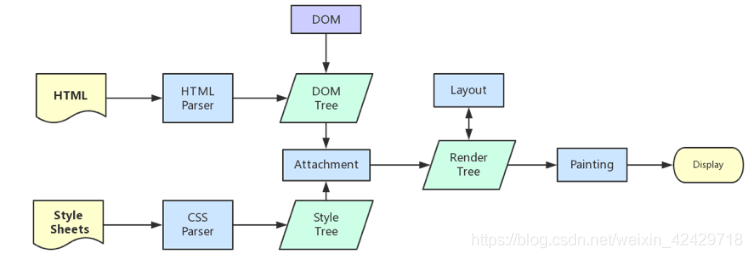### 考察 CSS ​

#### css 样式优先级，以及渲染过程 ​

• 内联样式(例如, style="...")
• ID 选择器(例如, #example)
• 类型选择器(例如, h1)、伪元素(例如, ::before)
• 继承的样式

#### 下面样式是怎样渲染的 ​

javascript
``````.container .inner div{
width: 100px;
height: 100px;
}``````
``````.container .inner div{
width: 100px;
height: 100px;
}``````

#### 下面代码，父级元素 container 高度是多少？ ​

javascript
``````<div class="container">
<div class="inner"/>
<div class="inner"/>
</div>

.container {
border: 1px;
}
.inner {
margin: 10px;
height=width: 10px;
}``````
``````<div class="container">
<div class="inner"/>
<div class="inner"/>
</div>

.container {
border: 1px;
}
.inner {
margin: 10px;
height=width: 10px;
}``````

javascript
``````<!DOCTYPE html>
<html lang="en">
<meta charset="UTF-8">
<meta name="viewport" content="width=device-width, initial-scale=1.0">
<title>Document</title>
<style>
.container {
border: 1px;
}
.inner1 {
margin: 10px;
height: 10px;
width: 10px;
background: red;
}
.inner2 {
margin: 10px;
height: 10px;
width: 10px;
background: blue;
}
</style>
<body>
<div class="container">
<div class="inner1"></div>
<div class="inner2"></div>
</div>
</body>
</html>``````
``````<!DOCTYPE html>
<html lang="en">
<meta charset="UTF-8">
<meta name="viewport" content="width=device-width, initial-scale=1.0">
<title>Document</title>
<style>
.container {
border: 1px;
}
.inner1 {
margin: 10px;
height: 10px;
width: 10px;
background: red;
}
.inner2 {
margin: 10px;
height: 10px;
width: 10px;
background: blue;
}
</style>
<body>
<div class="container">
<div class="inner1"></div>
<div class="inner2"></div>
</div>
</body>
</html>``````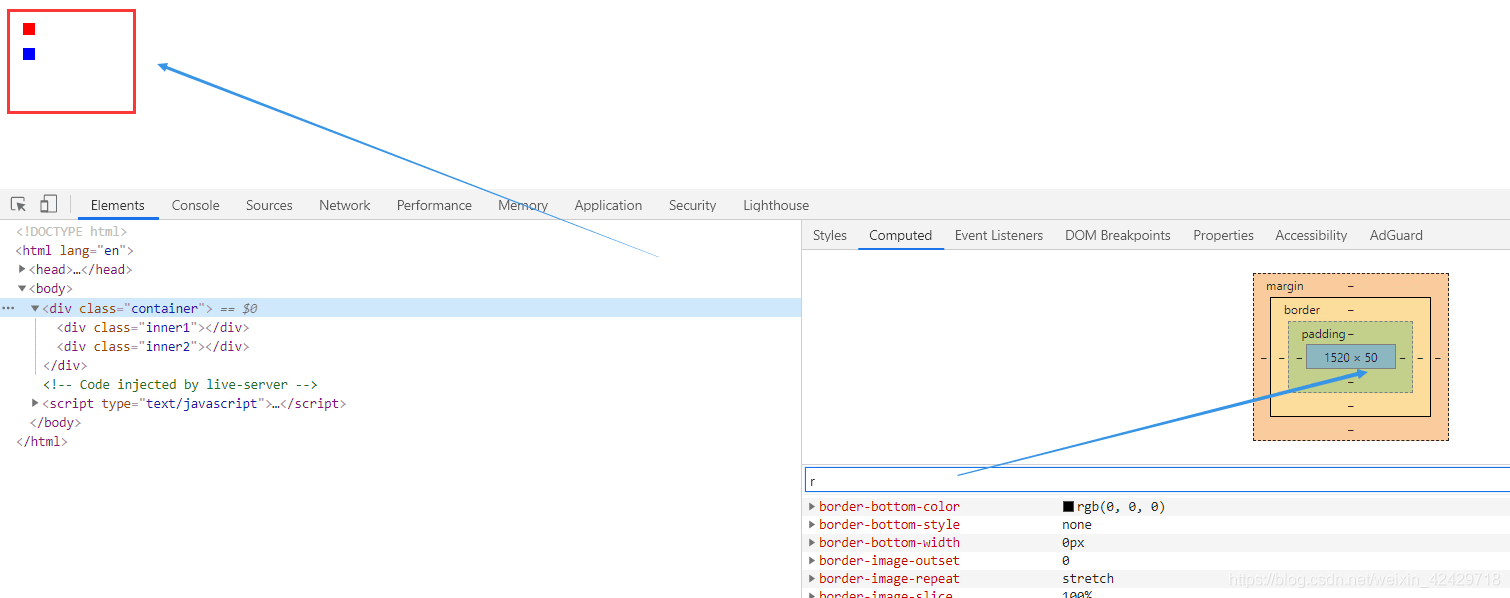javascript
``````<div class="container">
<div class="inner"/>
<div class="inner"/>
</div>

.container {
border: 1px;
}
.inner {
float: left;
margin: 10px;
height=width: 10px;
}``````
``````<div class="container">
<div class="inner"/>
<div class="inner"/>
</div>

.container {
border: 1px;
}
.inner {
float: left;
margin: 10px;
height=width: 10px;
}``````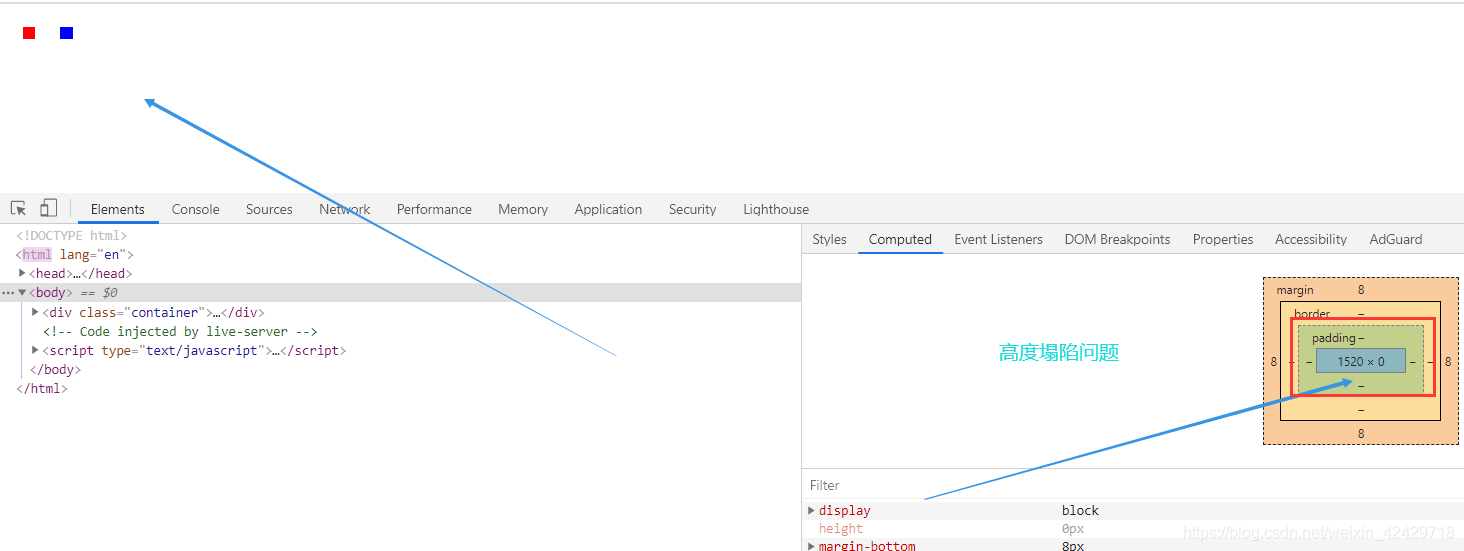css
``````.container {
border: 1px;
overflow: hidden;
}``````
``````.container {
border: 1px;
overflow: hidden;
}``````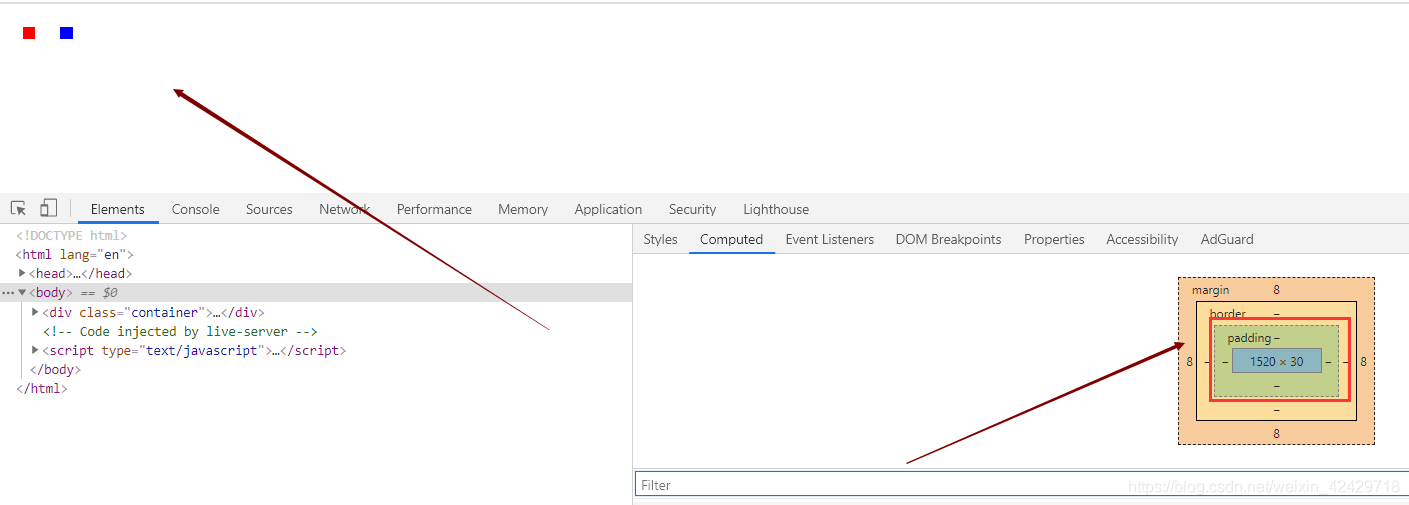### 考察 JS ​

#### 下面代码会输出什么？（考察变量提升） ​

##### 第一步 ​
javascript
``console.log(a);``
``console.log(a);``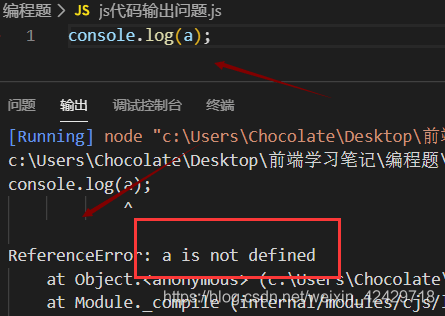##### 第二步 ​
javascript
``````console.log(a);
var a = 1;``````
``````console.log(a);
var a = 1;``````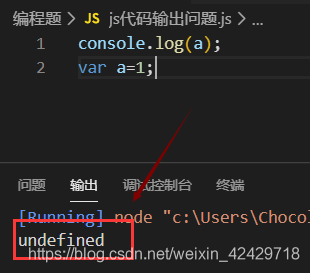##### 第三步 ​
javascript
``````console.log(a);
var a = 1;
function a() {}``````
``````console.log(a);
var a = 1;
function a() {}``````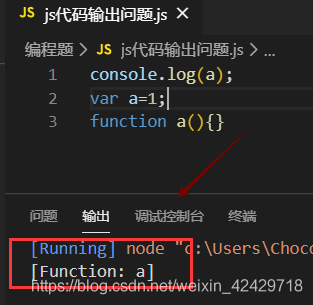##### 第四步 ​
javascript
``````console.log(a);
var a = 1;
function a() {}
a = 2;``````
``````console.log(a);
var a = 1;
function a() {}
a = 2;``````

##### 第五步 ​
javascript
``````console.log(a);
var a = 1;
function a() {}
a = 2;
a();``````
``````console.log(a);
var a = 1;
function a() {}
a = 2;
a();``````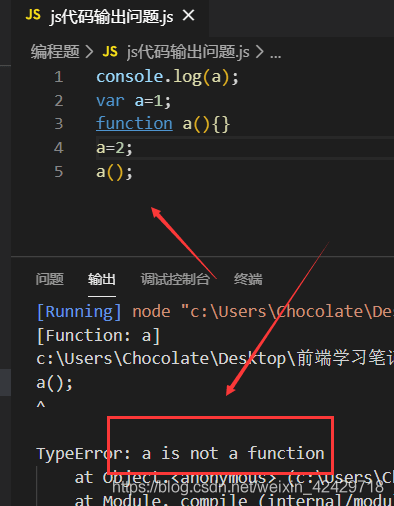#### 下面代码会输出什么？（考察事件循环） ​

##### 第一步 ​
javascript
``````setTimeout(() => {
console.log(1);
}, 0);
console.log(2);
// 输出结果：2 1``````
``````setTimeout(() => {
console.log(1);
}, 0);
console.log(2);
// 输出结果：2 1``````
##### 第二步 ​
javascript
``````setTimeout(() => {
console.log(1);
}, 0);
console.log(2);
new Promise((resolve) => {
console.log(3);
resolve(4);
}).then((val) => console.log(val));
// 输出结果：2 3 4 1``````
``````setTimeout(() => {
console.log(1);
}, 0);
console.log(2);
new Promise((resolve) => {
console.log(3);
resolve(4);
}).then((val) => console.log(val));
// 输出结果：2 3 4 1``````
##### 第三步 ​
javascript
``````document.body.addEventListener(
"click",
() => {
Promise.resolve().then(() => console.log(1));
console.log(2);
},
false
);

"click",
() => {
Promise.resolve().then(() => console.log(3));
console.log(4);
},
false
);``````
``````document.body.addEventListener(
"click",
() => {
Promise.resolve().then(() => console.log(1));
console.log(2);
},
false
);

"click",
() => {
Promise.resolve().then(() => console.log(3));
console.log(4);
},
false
);``````

javascript
``document.body.click();``
``document.body.click();``

### 算法题 ​

#### 你了解回文字符串吗？例如 abba，写一下代码吧（不限语言） ​

cpp
``````#include<bits/stdc++.h>
using namespace std;
int main(){
string str;
cin>>str;
int len=str.length();
int mid=(len+1)/2;
string ans1=str.substr(0,mid); //取前半段字符串
if(len&1) mid--;
string ans2=str.substr(mid,len-mid); //取后半段字符串
reverse(ans2.begin(),ans2.end()); //取反
if(ans1==ans2) cout<<1<<endl;
else cout<<0<<endl;
return 0;
}``````
``````#include<bits/stdc++.h>
using namespace std;
int main(){
string str;
cin>>str;
int len=str.length();
int mid=(len+1)/2;
string ans1=str.substr(0,mid); //取前半段字符串
if(len&1) mid--;
string ans2=str.substr(mid,len-mid); //取后半段字符串
reverse(ans2.begin(),ans2.end()); //取反
if(ans1==ans2) cout<<1<<endl;
else cout<<0<<endl;
return 0;
}``````

#### 那你可以求出字符串中最长回文子串吗？例如给你 abbac，得到 abba ​

cpp
``````#include<bits/stdc++.h>
#define endl '\n'
using namespace std;
const int maxn=1e3+5;
int p[maxn];
int main(){
string str;
while(cin>>str){
string s="\$";//第一步:预处理，将原字符串转换为新字符串
for(int i=0;i<str.length();i++)
s+="#",s+=str[i];
s+="#@"; //尾部再加上字符@，将偶数长度变为奇数长度
int n=s.length();
int id=0,mx=0; //初始化中心位置和最右端位置
int maxlen=-1,idx=0;
for(int j=1;j<n-1;j++){
p[j]=mx>j? min(p[2*id-j],mx-j):1;
while(s.at(j+p[j]) == s.at(j-p[j])) //向左右两边拓展
p[j]++;
if(mx<p[j]+j){ //如果超过右边界，进行更新
mx=p[j]+j;
id=j;
}
if(maxlen<p[j]-1){ //更新最大长度和中心索引位置
maxlen=p[j]-1;
idx=j;
}
}
int start=(idx-maxlen)/2;  //求起始点索引
string ans=str.substr(start,maxlen); //字符串截取得到最长回文子串
cout<<ans<<endl;
}
return 0;
}``````
``````#include<bits/stdc++.h>
#define endl '\n'
using namespace std;
const int maxn=1e3+5;
int p[maxn];
int main(){
string str;
while(cin>>str){
string s="\$";//第一步:预处理，将原字符串转换为新字符串
for(int i=0;i<str.length();i++)
s+="#",s+=str[i];
s+="#@"; //尾部再加上字符@，将偶数长度变为奇数长度
int n=s.length();
int id=0,mx=0; //初始化中心位置和最右端位置
int maxlen=-1,idx=0;
for(int j=1;j<n-1;j++){
p[j]=mx>j? min(p[2*id-j],mx-j):1;
while(s.at(j+p[j]) == s.at(j-p[j])) //向左右两边拓展
p[j]++;
if(mx<p[j]+j){ //如果超过右边界，进行更新
mx=p[j]+j;
id=j;
}
if(maxlen<p[j]-1){ //更新最大长度和中心索引位置
maxlen=p[j]-1;
idx=j;
}
}
int start=(idx-maxlen)/2;  //求起始点索引
string ans=str.substr(start,maxlen); //字符串截取得到最长回文子串
cout<<ans<<endl;
}
return 0;
}``````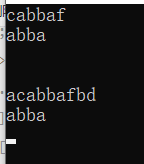## 总结 ​

• 无需惧怕算法题，难度真的没有 `acm` 那个高度
• 将知识重新捡起来，不要在要面试的时候，才临时抢记知识
• 安静地努力，每篇文章写完后就要消化，不要没弄懂就发布
• 文章不在于多，而在于精，将每篇文章都要认真书写，让读者都能一读即懂

javascript
``学如逆水行舟，不进则退``
``学如逆水行舟，不进则退``